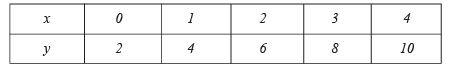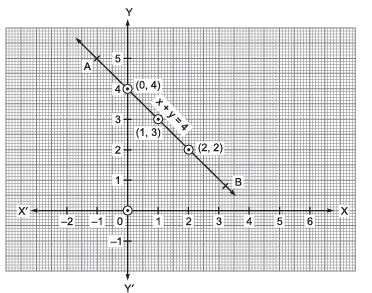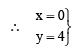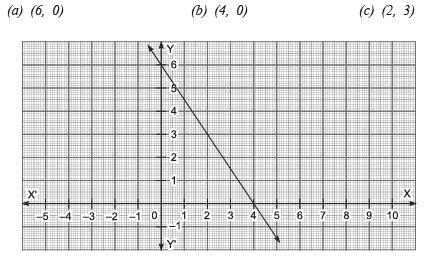# Very Short Answer Type Questions: Linear Equations in Two Variables Notes | Study Mathematics (Maths) Class 9 - Class 9

## Class 9: Very Short Answer Type Questions: Linear Equations in Two Variables Notes | Study Mathematics (Maths) Class 9 - Class 9

The document Very Short Answer Type Questions: Linear Equations in Two Variables Notes | Study Mathematics (Maths) Class 9 - Class 9 is a part of the Class 9 Course Mathematics (Maths) Class 9.
All you need of Class 9 at this link: Class 9

Question 1. Show that x = 1, y = 3 satisfy the linear equation 3x – 4y + 9 = 0.
Solution: We have 3x – 4y + 9 = 0
Putting x = 1, y = 3, we get
L.H.S. = 3(1) – 4(3) + 9 = 3 – 12 + 9
= 12 – 12 = 0 = R.H.S.
Since, L.H.S. = R.H.S.
∴ x = 1 and y = 3 satisfy the given linear equation.

Question 2. Write whether the following statements are True or False? Justify your answers.
(i) ax + by + c, where a, b and c are real numbers, is a linear equation in two variables.

(ii) A linear equation 2x + 3y = 5 has a unique solution.

(iii) All the points (2, 0), (–3, 0), (4, 2) and (0, 5) lie on the x-axis.

(iv) The line parallel to y-axis at a distance 4 units to the left of y-axis is given by the equation x = –4.

(v) The graph of the equation y = mx + c passes through the origin.
Solution.

(i) False. [Because ax + by + c = 0 is a linear equation in two variables if both ‘a‘ and ‘b’ are non-zero.]

(ii) False. [Because a linear equation in two variables has infinitely many solutions.]

(iii) False. [Because the points (2, 0) and (–3, 0) lie on the x-axis, (0, 5) lie on the y-axis whereas the point (4, 2) lies in the first quadrant.]

(iv) True.

(v) False. [Because the point (0, 0) i.e., x = 0 + y = 0 does not satisfy the equation]

Question 3. Write whether the following statement is True or False? Justify your answer.
The coordinates of points given in the table:Represent some of the solutions of the equation 2x + 2 = y.
Solution. True. [Since, on looking at the given coordinates, we observe that each y-coordinate is two units more than double the x-coordinate.]

Question 4. Look at the following graphical representation of an equation. Which of the points (0, 0) (0, 4) or (–1, 4) is a solution of the equation?Solution: Since, the point (0, 4) lies on the line.is a solution of the equation.
.

Question 5. Look at the following graphical representation of an equation. Which of the following is not its solution?Solution: The point (6, 0) does not lie on the graph.
∴ The point (6, 0) is not the solution of the equation.

The document Very Short Answer Type Questions: Linear Equations in Two Variables Notes | Study Mathematics (Maths) Class 9 - Class 9 is a part of the Class 9 Course Mathematics (Maths) Class 9.
All you need of Class 9 at this link: Class 9Use Code STAYHOME200 and get INR 200 additional OFF

## Mathematics (Maths) Class 9

73 videos|351 docs|110 tests

Track your progress, build streaks, highlight & save important lessons and more!

,

,

,

,

,

,

,

,

,

,

,

,

,

,

,

,

,

,

,

,

,

;